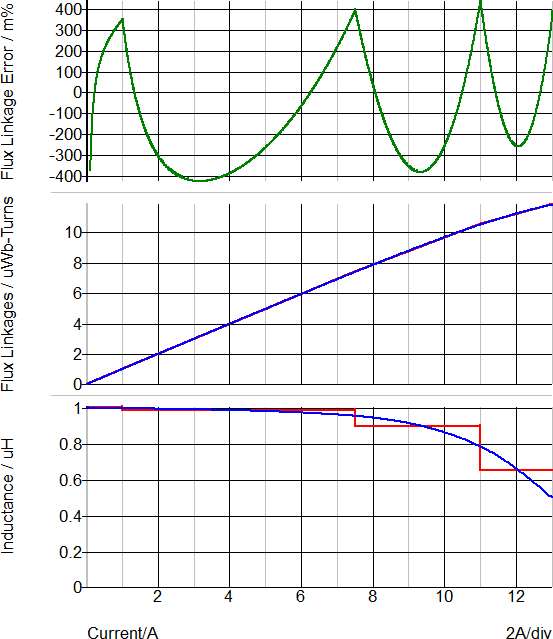# Multi-Level Lossy PWL Inductor (Version 8.0+)

The Multi-Level PWL Inductor model has the same model levels as the Multi-Level Lossy Inductor, and other than the internal inductor, the models are identical. The inductor internal to the PWL inductor can have up to 10 segments and the inductance is defined on a Flux Linkage vs. Current plane. As the model level increases, so does the model complexity. This inductor is available with Version 8.0 or later.

In this topic:

 Model Name: Multi-Level Lossy PWL Inductor Simulator: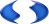This device is compatible with both the SIMetrix and SIMPLIS simulators. Parts Selector Menu Location: Magnetics > Inductors > Multi-Level Lossy PWL Inductor (Version 8.0+) Symbol Library: passives.lb Model File: simplis_param.lb Subcircuit Name: MULTI_LEVEL_LOSSY_PWL_IND_QTY Symbol: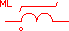Multiple Selections: Multiple devices can be selected and edited simultaneously.

## Editing the Multi-Level Lossy PWL Inductor

To configure the Multi-Level lossy PWL Inductor, follow these steps:

1. Double click the symbol on the schematic to open the editing dialog to the Parameters tab.
2. Choose a model level, and then make the appropriate changes to the fields described in the table below the image.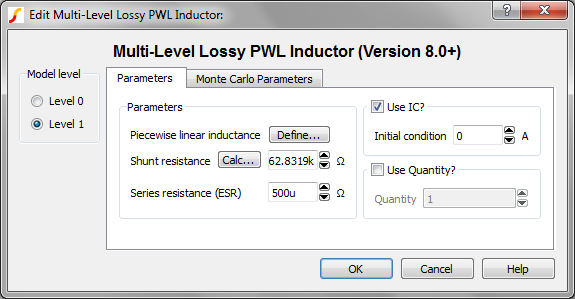Label Applicable Model(s) Units Description Piecewise linear inductance 0,1 H Inductance parameters. SIMPLIS uses Piecewise linear (PWL) modeling techniques to model non-linear devices, which are defined using a table of X, Y points. Click Define... to open the table editor to define Current and Flux Linkagespoints. To insert rows in the table, select the number of rows you want to insert and press the insert key. To delete rows, select those you want to delete and press the delete key. Shunt resistance 0, 1 Ω The shunt resistance in parallel with the inductor. This resistance is included to limit the maximum frequency response of the inductor, which maximizes the simulation speed. Click Calc... to calculate this value. Series resistance (ESR) 1 Ω The equivalent series resistance (ESR) Use IC? 0,1 n/a If checked, the model uses the specified Initial condition. If unchecked, the inductor is a short circuit at time=0. Initial condition 0,1 A Initial current at time=0 Use Quantity? 0,1 n/a If checked, the model uses the specified Quantity of inductors in parallel. Quantity 0,1 n/a Number of inductors in parallel. Note: To maximize simulation speed when using SIMPLIS, place a single symbol and specify a value for this parameter instead of placing multiple inductors.

The Monte Carlo Parameters are shown and explained below.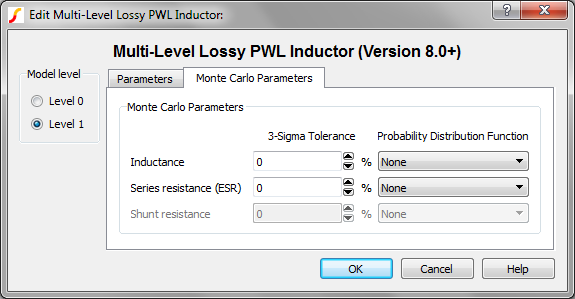Label Applicable Model(s) Units Description Inductance 0,1 % 3-sigma tolerance for the inductance value Shunt resistance 0,1 % 3-sigma tolerance for the shunt resistance in parallel with the inductor. This resistance is included to limit the maximum frequency response of the inductor, which maximizes the simulation speed. Probability Distribution Function n/a n/a The choices for this function for each available parameter are the following: None Gauss Truncated Gauss Uniform Worst Case

## Multi-Level PWL Lossy Inductor Model Levels

The Multi-Level Inductor has two levels: 0, 1. As the model level increases, so does the model complexity; and, as a rule, simulation times also increase.

### Level 0 Model

Level 0 models an inductor with a parallel shunt resistance.

Level 0 models these circuit elements Level 0 Schematic
 L_IND PWL Inductance R_SHUNT Inductor shunt resistor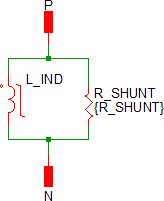### Level 1 Model

Level 1 models an inductor with a parallel shunt resistance and an equivalent series resistance (ESR).

Level 1 models these circuit elements Level 1 Schematic
 L_IND PWL Inductance R_SHUNT Inductor shunt resistor ESR Series resistance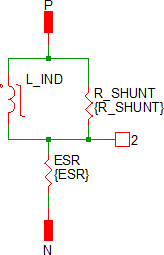### Level 2 Model

The Level 2 model is electrically identical to the level 1 model. The only difference between the two model levels is that the level 2 model is created by the SIMPLIS Magnetic Design Module (MDM). This model represents a physical magnetic design, with a specific core, winding, bobbin, etc. For this reason, the model doesn't implement the Monte Carlo parameters seen on the other model levels.

## Quantity Implementation

The Multi-Level Inductor model has two quantity parameters, USE_QTY and QTY, which specify the number of inductors in parallel. Configuring these parameters minimizes the number of reactive circuit elements in the model and, therefore, provides a maximum simulation speed.

The implementation of the quantity parameter uses a "DC Transformer" technique where the inductor's terminal current is multiplied by a constant using a Current Controlled Current Source (CCCS). This technique effectively divides all resistance and inductance values by the quantity parameter (QTY). This circuitry is added if the USE_QTY parameter is set to 1.

A schematic of the quantity implementation for a Level 0 Multi-Level PWL Inductor is shown below: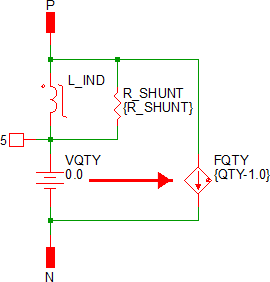## Example

An example schematic can be downloaded here: simplis_008_multi_level_pwl_inductor_example.sxsch.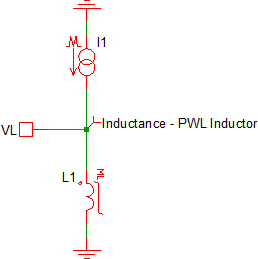Shown below is a four segment inductor taken from a data sheet curve of a typical 1uH, 10A power inductor, these are the default parameters for the symbol: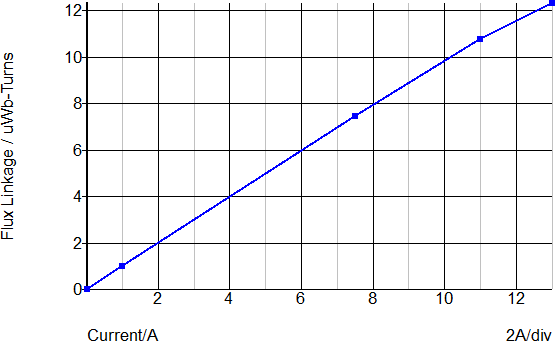These Flux Linkage-Current point pairs are input on a PWL table dialog, which is opened by clicking on the "Define..." button: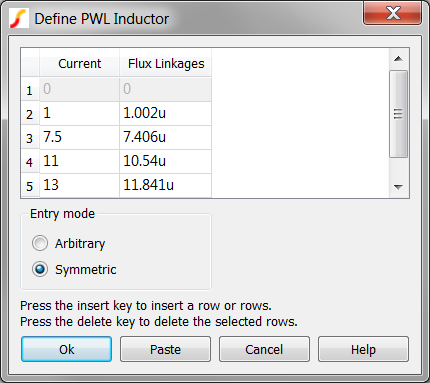## Waveforms

This schematic has the datasheet curve points stored in a script located in the schematic's F11 window. After pressing F9 to run the schematic, the data sheet curves for the inductance and flux linkage are automatically generated. The script also generates the percentage error between the datasheet flux linkage and the PWL inductor flux linkage curves. The maximum flux linkage error is approximately +/-0.4%. More PWL segments could be added to reduce this error, at the expense of longer simulation times. The data sheet curve for this inductor was digitized using the Tools ▶ Digitise Data Sheet Curve...